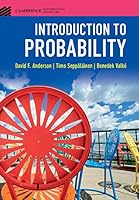# Introduction to Probability## Book Description

This classroom-tested textbook is an introduction to probability , with the right balance between precision, probabilistic intuition, and concrete applications. Introduction to Probability covers the material precisely, while avoiding excessive technical details. After introducing the basic vocabulary of randomness, including events, probabilities, and random variables, the text offers the reader a first glimpse of the major theorems of the subject: the of large numbers and the central limit theorem. The important probability distributions are introduced organically as they arise from applications. The discrete and continuous sides of probability are treated together to emphasize their similarities. Intended for students with a background, the text teaches not only the nuts and bolts of probability theory and how to solve specific problems, but also why the methods of solution work.